The NLMIXED Procedure

Example 70.7 Overdispersion Hierarchical Nonlinear Mixed Model

This example describes the overdispersion hierarchical nonlinear mixed model that is given in Ghebretinsae et al. (2013). In this experiment, 24 rats are divided into four groups and receive a daily oral dose of 1,2-dimethylhydrazine dihydrochloride in three dose levels (low, medium, and high) and a vehicle control. In addition, an extra group of three animals receive a positive control. All 27 animals are euthanized 3 hours after the last dose is administered. For each animal, a cell suspension is prepared, and from each cell suspension, three replicate samples are prepared for scoring. Using a semi-automated scoring system, 50 randomly selected cells from each sample are scored per animal for DNA damage. The comet assay methodology is used to detect this DNA damage. In this methodology, the DNA damage is measured by the distance of DNA migration from the perimeter of the comet head to the last visible point in the tail. This distance is defined as the tail length and is observed for the 150 cells from each animal. The data are available in the Sashelp library. In order to use the dose levels as regressors in PROC NLMIXED, you need to create indicator variables for each dose level. The following DATA step creates indicator variables for the dose levels:

data comet;
set sashelp.comet;
L = 0; M = 0; H = 0; PC = 0;
if (dose = 1.25) then L  = 1;
if (dose = 2.50) then M  = 1;
if (dose = 5.00) then H  = 1;
if (dose = 200)  then PC = 1;
run;


Ghebretinsae et al. (2013) suggest a Weibull distribution to model the outcomes (tail lengths). Note that the data exhibit the nested nature because of the three replicate samples from each cell. Also, the 50 cells from each rat are correlated. To account for the dependency of these 150 correlated cells from each rat, two levels of normally distributed nested random effects are used. Let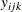be the tail length that is observed in the kth cell from the jth sample of the ith rat. Then the suggested two-level nested model can be described as follows: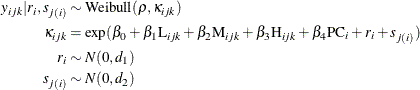This model is referred to as the nested Weibull model in the rest of the example. The parameters of the Weibull distribution,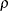and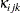, are known as the shape and scale parameters, respectively. Based on this model specification, the conditional expectation of the response variable, given the random effects, is a nonlinear function of fixed and random effects. The nonlinear relationship can be written as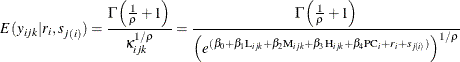These expected values can be obtained using the PREDICT statement in PROC NLMIXED, and they are useful for validating the model fitting. The specified nested Weibull model can be fitted using the following PROC NLMIXED statements:

proc nlmixed data = comet;
parms b0 = -25  b1 = -10 b2 = -10 b3 = -10 b4 = -10 rho = 10;
bounds sd1 >=0, sd2 >=0;
mu = b0+ b1*L+ b2*M+ b3*H+ b4*PC + rateff + sampeff;
term = (length**rho)*exp(mu);
llike = log(rho) + (rho-1)*log(length) + mu - term;
model length ~ general(llike);
random ratEff ~ normal(0,sd1) subject = rat;
random sampEff ~ normal(0,sd2) subject = sample(rat);
predict gamma(1+(1/rho))/(exp(mu)**(1/rho)) out = p1;
run;


Note that the two-parameter Weibull density function is given by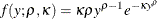This implies that the log likelihood is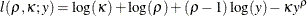A general log-likelihood specification is used in the MODEL statement to specify the preceding log of the Weibull density. The linear combination of the parameters, bto b, and the two random effects, RatEff and SampEff, is defined using the linear predictor MU in the first SAS programming statement. Then, the next two SAS programming statements together define the log of the Weibull density function. The first RANDOM statement defines the first level of the random effect,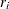, by using RatEff. Then, the second RANDOM statement defines the second level of the random effect,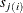, nested within the first level by using SampEff. Both random-effects distributions are specified as normal with mean 0 and variance sd1 and sd2 for the first and second levels, respectively. The selected output from this model is shown in Output 70.7.1, Output 70.7.2, and Output 70.7.3.

Output 70.7.1: Model Specification for Nested Weibull Model

The NLMIXED Procedure

Specifications
Data Set WORK.COMET
Dependent Variable Length
Distribution for Dependent Variable General
Random Effects rateff, sampeff
Distribution for Random Effects Normal, Normal
Subject Variables Rat, Sample
Optimization Technique Dual Quasi-Newton

The "Specifications" table shows that RatEff is the first-level random effect, specified by the subject variable Rat, and it follows a normal distribution (Output 70.7.1). Similarly, SampEff is the second-level random effect, nested in RatEff and specified by the subject variable Sample, and it follows a normal distribution.

Output 70.7.2: Dimensions Table for Nested Weibull Model

Dimensions
Observations Used 4050
Observations Not Used 0
Total Observations 4050
Subjects [Rat] 27
Max Obs per Subject 150
Subjects [Sample] 81
Max Obs per Subject 50
Parameters 8

The "Dimensions" table indicates that 4,050 observations are used in the analysis (Output 70.7.2). It also indicates that there are 27 rats and that 81 samples are nested within the rats. As explained earlier, three samples are selected randomly from each rat, so a total of 81 (27 times 3) samples are used in the analysis.

Output 70.7.3: Parameter Estimates for Nested Weibull Model

Parameter Estimates
Parameter Estimate Standard
Error
DF t Value Pr > |t| 95% Confidence Limits Gradient
b0 -15.6346 0.2627 79 -59.52 <.0001 -16.1575 -15.1117 -0.21455
b1 -4.4912 0.2345 79 -19.15 <.0001 -4.9581 -4.0244 -0.12563
b2 -4.5865 0.2186 79 -20.98 <.0001 -5.0216 -4.1514 0.20926
b3 -4.8188 0.2188 79 -22.02 <.0001 -5.2543 -4.3833 0.088666
b4 -3.4834 0.2664 79 -13.08 <.0001 -4.0136 -2.9532 -0.18096
rho 4.9558 0.05881 79 84.27 <.0001 4.8388 5.0729 -1.18121
sd1 0.02454 0.04601 79 0.53 0.5953 -0.06704 0.1161 -0.75888
sd2 0.3888 0.07106 79 5.47 <.0001 0.2473 0.5302 -0.79003

The "Parameter Estimates" table list the maximum likelihood estimates of the parameters along with their standard errors (Output 70.7.3). The estimates are fairly close to the estimates that are given in Ghebretinsae et al. (2013), who use a gamma distribution for the Sample random effects instead of the normal distribution as in the preceding model. Note that the parameters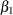,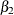, and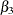represent the effects of low, medium, and high doses, respectively, when compared to the vehicle control. Similarly,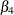is the parameter that corresponds to the effect of positive control when compared to vehicle control. The p-value indicates that the point estimates of all these parameters,to, are significant; this implies that the toxicity of 1,2-dimethylhydrazine dihydrochloride is significant at different dose levels.

In the nested Weibull model, the scale parameter,, is random because it is a function of random effects. This implies that the model assumes that each observation is drawn from a different Weibull distribution. Molenberghs et al. (2010) indicate that this way of modeling leads to an overdispersed Weibull model. Ghebretinsae et al. (2013) use the random-effects mechanism to account for this overdispersion. The overdispersed Weibull model can be written as follows: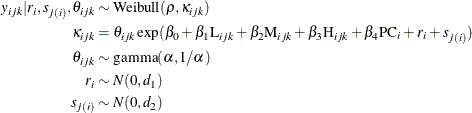This model is referred to as the nested Weibull overdispersion model in the rest of this example. Again, in this model, the shape parameter,, is the function of the normally distributed random effects,and, along with other random effects,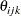. Further, the model assumes thatfollow a gamma distribution. Note that the random effects,and, account for the cluster effect of the observations from the same sample that is nested within a rat, whereasaccount for the overdispersion in the data. Molenberghs et al. (2010) integrate out the overdispersion random effects,, from the joint distribution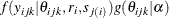and provide the conditional density of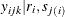. The conditional density follows: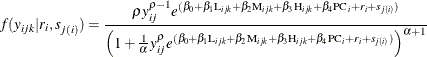Again, in this case, the conditional expectation of the response variable, given the random effects, is a nonlinear function of the fixed and random effects. Molenberghs et al. (2010) provide this nonlinear relationship as follows: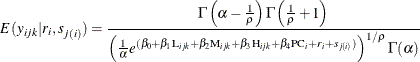As indicated previously, these values are useful for validating the fit of the model, and they can be obtained using a PREDICT statement in PROC NLMIXED. Using the preceding conditional distribution of response, given the random effects and the random-effects distribution, PROC NLMIXED fits the two-level nested Weibull overdispersion model by using the following statements:

ods select ParameterEstimates;
proc nlmixed data = comet;
parms b0 = -25  b1 = -10 b2 = -10 b3 = -10 b4 = -10 alpha = 1 rho = 10;
bounds sd1 >=0, sd2 >=0;
mu = b0+ b1*L+ b2*M+ b3*H+ b4*PC + rateff + sampeff;
num = rho*((length)**(rho-1))*exp(mu);
den = (1+(((length)**rho)*exp(mu))/alpha)**(alpha+1);
llike = log(num/den);
model length ~ general(llike);
random rateff ~ normal(0,sd1) subject = rat;
random sampeff ~ normal(0,sd2) subject = sample(rat);
predict (gamma(alpha-(1/rho))*gamma(1+(1/rho)))
/(gamma(alpha)*((exp(mu)/alpha)**(1/rho))) out = p2;
run;


The parameter estimates that are obtained from the nested Weibull overdispersion model are given in Output 70.7.1. The "Parameter Estimates" table indicates that the point estimates are still significant, as they are in the previous model in which the overdispersion is not taken into consideration. So the same conclusion about the dose levels holds: the toxicity of 1,2-dimethylhydrazine dihydrochloride is significant at different dose levels.

Output 70.7.4: Parameter Estimates for Nested Weibull Overdispersion Model

The NLMIXED Procedure

Parameter Estimates
Parameter Estimate Standard
Error
DF t Value Pr > |t| 95% Confidence Limits Gradient
b0 -31.0041 0.8155 79 -38.02 <.0001 -32.6272 -29.3810 -0.27124
b1 -11.9102 0.5159 79 -23.09 <.0001 -12.9371 -10.8833 -0.00736
b2 -12.1194 0.5173 79 -23.43 <.0001 -13.1492 -11.0897 0.095998
b3 -12.5516 0.5217 79 -24.06 <.0001 -13.5900 -11.5132 0.29527
b4 -9.6307 0.5554 79 -17.34 <.0001 -10.7362 -8.5251 -0.12589
alpha 0.8925 0.05047 79 17.68 <.0001 0.7920 0.9930 1.84146
rho 10.7188 0.2792 79 38.39 <.0001 10.1632 11.2745 -0.04855
sd1 0.1908 0.1549 79 1.23 0.2217 -0.1175 0.4990 0.49983
sd2 0.7926 0.1666 79 4.76 <.0001 0.4609 1.1242 -0.04111

Recall that, from both models, the conditional expected values of the response variable, given the random effects, are obtained using the PREDICT statement. These predicted values are stored in the data sets p1 and p2 for the nested Weibull and nested Weibull overdispersion models, respectively. Because, in this example, the regressor variables are only indicators, the prediction values for all the observations from the same sample that is nested within a rat are equal. Further, compute the average predicted value for each sample that is nested within a rat for both models. These values predict the average tail length of each sample that is nested within a rat based on the fitted model. In addition, compute the observed sample averages of the responses from each cluster, which can be viewed as the crude estimate of the average population tail length for each cluster. The following statements combine all these average tail lengths, both observed and predicted, from the two fitted models into a single data set:

proc means data = p1 mean noprint;
class sample;
var pred;
output out = p11 (keep = sample weibull_mean)mean = weibull_mean;
run;

proc means data = p2 mean noprint;
class sample;
var pred;
output out = p21 (keep = sample weibull_gamma_mean)mean = weibull_gamma_mean;
run;

proc means data = comet mean noprint;
class sample;
var length;
id dose rat;
output out = p31(keep = dose rat sample observed_mean) mean = observed_mean;
run;

data average;
merge p11 p21 p31;
by sample;
if sample = . then delete;
label observed_mean = "Observed Average";
label weibull_gamma_mean = "Weibull Gamma Average";
label weibull_mean = "Weibull Average";
label sample = "Sample Index";
if dose = 0 then dosage = "0 Vehicle Control ";
if dose = 1.25 then dosage = "1 Low";
if dose = 2.50 then dosage = "2 Medium";
if dose = 5.00 then dosage = "3 High";
if dose = 200  then dosage = "4 Positive Control";
run;


In order to validate the fit of the models, the observed average tail length values are plotted along with predicted average tail lengths from each model that is fitted using PROC NLMIXED. Moreover, the plots are created for each dosage level by using a PANELBY statement in PROC SGPANEL. The code follows:

proc sgpanel data = average;
panelby dosage/onepanel layout = columnlattice novarname uniscale = row;
rowaxis values=(10 to 80 by 5) label="Average Tail Length";
series x = sample y = observed_mean;
series x = sample y = weibull_mean;
series x = sample y = weibull_gamma_mean;
run;


The resulting plots are given in Output 70.7.5.

Output 70.7.5: Comparison of Nested Weibull Model and Nested Weibull Overdispersion Model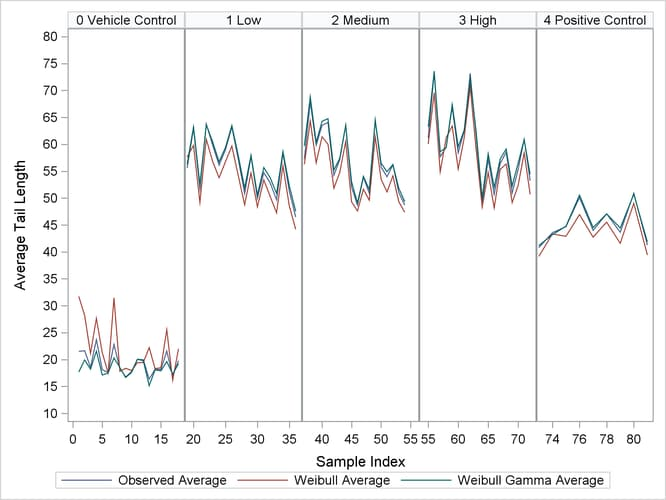You can see from the plots that the observed averages of the tail lengths from each sample that is nested within a rat are closer to the predicted averages of the nested Weibull overdispersion model than to the predicted averages of the nested Weibull model.